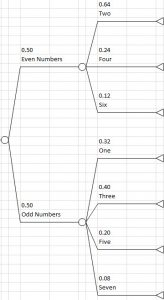# TreePlan Limit of Five Branches per Node

The TreePlan decision tree add-in is limited to a maximum of five branches per node.

If you need to represent a set of alternatives or a set of outcomes with more than five elements, a workaround is to use two sets of branches, one following the other.

The following example shows two ways to represent a set of seven outcomes with the following probability assignment.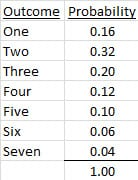One way is to use a first set of branches with five branches: one branch for each of the first four outcomes, and a fifth branch for the event More Than Four.

P(More Than Four) = P(Five) + P(Six) + P(Seven) = 0.10 + 0.06 + 0.04 = 0.20

The second set of three branches following More Than Four uses the probability for each outcome Five, Six, or Seven, conditional on the event More Than Four.

For example, P(Five | More Than Four) = P(Five) / P(More Than Four)

= 0.10 / (0.10 + 0.06 + 0.04) = 0.10 / 0.20 = 0.50

The following tree diagram shows the calculation formulas for each probability.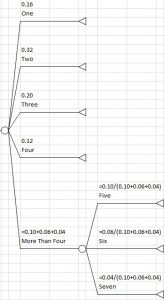The following tree diagram shows how the probabilities are displayed.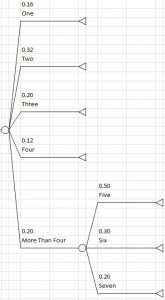The path combining the product of the probabilities on the branch More Than Four and the branch Five yields the probability of the original outcome:

P(More Than Four) * P(Five | More Than Four) = 0.20 * 0.50 = 0.10 = P(Five)

A second way to represent the same seven outcomes is to use a first set of branches with two outcomes: Even Numbers and Odd Numbers.

The seven outcomes and probabilities may be grouped as shown in the following table.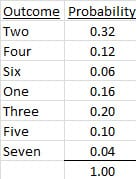The following tree diagram shows the calculation formulas for each probability.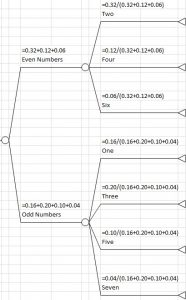The following tree diagram shows how the probabilities are displayed.